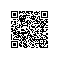# 古典加密算法-----置换密码

 置换密码算法的原理是不改变明文字符，只将字符在明文中的排列顺序改变，从而实现明文信息的加密。置换密码有时又称为换位密码。 矩阵换位法是实现置换密码的一种常用方法。它将明文中的字母按照给的顺序安排在一个矩阵中，然后用根据密钥提供的顺序重新组合矩阵中字母，从而形成密文。例如，明文为attack begins at five，密钥为cipher，将明文按照每行6列的形式排在矩阵中，形成如下形式： a t t a c k b e g i n s a t f i v e 根据密钥cipher中各字母在字母表中出现的先后顺序，给定一个置换： 1 2 3 4 5 6 f = 1 4 5 3 2 6 根据上面的置换，将原有矩阵中的字母按照第1列，第4列，第5列，第3列，第2列，第6列的顺序排列，则有下面形式： a a c t t k b i n g e s a i v f t e 从而得到密文：aacttkbingesaivfte

1. package cn.hdu.edu.encrypt;
2.
3.
4. /*
5.  * 古典密码算法之  置换算法
6.  * @author ni掌柜
7.  */
8. public class Change {
9.     //当加密或者解密成功时返回CRYPT_OK，失败时返回CRYPT_ERROR
10.     private final static int CRYPT_OK =     1;
11.     private final static int CRYPT_ERROR = 0;
12.     /*
13.      * @param initCode 没有加密前的字符串
14.      * @CRYPT_OK 加密成功
15.      * @CRYPT_ERROR 加密失败
16.      */
17.     public static int encrypt(String initCode)throws Exception{
18.     //用来输出加密后的字符
19.     StringBuilder sb = new StringBuilder();
20.
21.     //获取initCode的字符串长度
22.     int codeLength = initCode.length();
23.     //根据这个codeLength来确定要几行6列
24.     int rows = (int)Math.ceil(codeLength/6.0 );
25.
26.     //构造一个rows行6列的数组
27.     char[][] initChar = new char[rows];
28.     //存放initCode中的所有字符，包括空格
29.     for(int i = 0; i < initChar.length; i++ )
30.         for(int j=0; j < initChar.length; j++){
31.             //将对应字符放入这个数组中
32.             try{
33.                 initChar[i][j] = initCode.charAt(
34.                         (i ) * initChar.length + j
35.                      );
36.             }catch(Exception e){
37.                 //多出来的用@表示
38.                 initChar[i][j] = 64;
39.             }
40.         }
41.     //进行如下转换
42.     /*
43.      * 00 01 02 03 04 05
44.      * 10  11 12  13 14  15
45.      */
46.      // 1 4 5 3 2 6
47.
48.     //构造一个rows行6列的数组 作为目标
49.     char[][] targetChar = new char[rows];
50.     for(int i = 0; i < targetChar.length; i++ )
51.         for(int j=0; j < targetChar.length; j++){
52.             //第二列的字符是initChar中的第四列的字符
53.             if(j == 1 ){
54.                 targetChar[i][j] = initChar[i];
55.             }
56.             //第三列的字符是initChar中的第五列的字符
57.             else if(j == 2){
58.                 targetChar[i][j] = initChar[i];
59.             }
60.             //第四列的字符是initChar中的第三列的字符
61.             else if(j == 3){
62.                 targetChar[i][j] = initChar[i];
63.             }
64.             //第五列的字符是initChar中的第二列的字符
65.             else if(j == 4){
66.                 targetChar[i][j] = initChar[i];
67.             }
68.             //第一和六列的字符不变
69.             else{
70.                 targetChar[i][j] = initChar[i][j];
71.             }
72.         }
73.     //整合成一个密文
74.     //==================输出======================
75.     for(int i = 0; i < targetChar.length; i++ )
76.         for(int j=0; j < targetChar.length; j++){
77.             sb.append(targetChar[i][j] );
78.         }
79. //  ==================输出======================
80. //  for(int i = 0; i < initChar.length; i++ )
81. //      for(int j=0; j < initChar.length; j++){
82. //          System.out.print(targetChar[i][j] );
83. //      }
84.     //以下就是加密后的字符
85.     String targetCode = sb.toString();
86.     System.out.println(targetCode);
87.         return CRYPT_OK;
88.     }
89.
90.
91.
92.
93.
94.
95.
96.
97.     /*
98.      * @param targetCode 加密后的字符串
99.      * @CRYPT_OK 解密成功
100.      * @CRYPT_ERROR 解密失败
101.      */
102.     public static int decrypt(String targetCode)throws Exception{
103.
104.         //用来输出解密后的字符
105.     StringBuilder sb = new StringBuilder();
106.
107.     //获取targetCode的字符串长度
108.     int codeLength = targetCode.length();
109.     //根据这个codeLength来确定要几行6列
110.     int rows = (int)Math.ceil(codeLength/6.0 );
111.
112.     //构造一个rows行6列的数组
113.     char[][] targetChar = new char[rows];
114.     //存放targetCode中的所有字符，包括空格
115.     for(int i = 0; i < targetChar.length; i++ )
116.         for(int j=0; j < targetChar.length; j++){
117.             //将对应字符放入这个数组中
118.                 targetChar[i][j] = targetCode.charAt(
119.                         (i ) * targetChar.length + j
120.                      );
121.         }
122.
123.     //由于加密的时候是如下变换，现在要变回来
124.     /*
125.      * 00 01 02 03 04 05
126.      * 10  11 12  13 14  15
127.      */
128.      // 1 4 5 3 2 6
129.
130.     //构造一个rows行6列的数组 作为目标
131.     char[][] initChar = new char[rows];
132.     for(int i = 0; i < initChar.length; i++ )
133.         for(int j=0; j < initChar.length; j++){
134.             //init第二列的字符是targetChar中的第四列的字符
135.             if(j == 1 ){
136.                 initChar[i][j] = targetChar[i];
137.             }
138.             //第三列的字符是targetChar中的第五列的字符
139.             else if(j == 2){
140.                 initChar[i][j] = targetChar[i];
141.             }
142.             //第四列的字符是targetChar中的第三列的字符
143.             else if(j == 3){
144.                 initChar[i][j] = targetChar[i];
145.             }
146.             //第五列的字符是targetChar中的第二列的字符
147.             else if(j == 4){
148.                 initChar[i][j] = targetChar[i];
149.             }
150.             //第一和六列的字符不变
151.             else{
152.                 initChar[i][j] = targetChar[i][j];
153.             }
154.         }
155.
156.
157.     //整合成一个密文
158.     //==================输出======================
159.     for(int i = 0; i < initChar.length; i++ )
160.         for(int j=0; j < initChar.length; j++){
161.             sb.append(initChar[i][j] );
162.         }
163.
164. //  ==================输出======================
165. //  for(int i = 0; i < initChar.length; i++ )
166. //      for(int j=0; j < initChar.length; j++){
167. //          System.out.print(targetChar[i][j] );
168. //      }
169.
170.
171.     //以下就是init的字符
172.     String initCode = sb.toString();
173.     System.out.println(initCode);
174.         return CRYPT_OK;
175.     }
176.
177.
178. }使用钉钉扫一扫加入圈子
+ 订阅